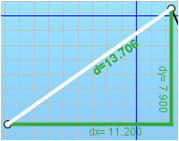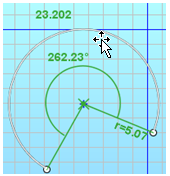# 2D sketcher: « Measure » tool

## Introduction

In the sketcher it is possible to measure :

• a distance between two points
• a line segment length
• a line arc length

The information is given graphically and also in the Exit .

## Measure point-point

The measure option for the distance between two existing points returns the following information:

D, Dx and DyIt is possible to consider a free point on the graph for the measure option. In this case, the information returned is only graphic, as the python command requires that a point should be a Flux geometry point.

## Measure of a segment

The measure option for a segment is a graphic shortcut to obtain the measure point (point related to the two extremity points of the segment). Therefore, the same information is obtained D, Dx and Dy.

## Measure of an arc

The measure option for an arc gives the following information:

Radius, Angle, curvilinear abscissa and the coordinates of the center pointThe coordinates of the center point are not graphically displayed to prevent graphic crowding.